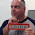## Monday, September 26, 2016

### The equivalent of MOVEA in all free RPGThere was a good question posted as a comment on the The different flavors of free format RPG post asking:

How do you move a array to field in free format. I did field1= arr1

In fixed format RPG I could use the Move Array operation code, MOVEA, to move data to and from arrays.

 ```01 D Array S 2 dim(10) 02 D Var1 S 20 03 D i S 2 0 04 C 1 do 10 i 05 C eval Array(i) = %char(i) 06 C enddo 07 C movea Array Var1 08 C movea 'AaBbCcDdEeFf'Array 09 C eval *inlr = *on ```

Alas, when free format calculations came out, in 2001 as part of V5R1, MOVEA was not given a free format equivalent.

I am sure there are different approaches to achieve the same end. This is just my way to perform the equivalent of the MOVEA. I am going to give my code in all free, but I will give a fixed format version of the definitions later.

 ```01 dcl-ds AllArray ; 02 Array char(2) dim(10) ; 03 end-ds ; 04 dcl-s Var1 char(20) ; 05 dcl-s i packed (2) ; 06 for i = 1 to %elem(Array) ; 07 Array(i) = %char(i) ; 08 endfor ; 09 Var1 = AllArray ; 10 AllArray = 'AaBbCcDdEeFfGgHhIi' ; ```

Lines 1: The array I am going to "MOVEA" needs to be in a data structure. In this example the data structure has the totally unimaginative name of AllArray.

Line 2: Here is the definition of the array. This is exactly the same way I would define a "standalone" array.

Line 3: End of the data structure.

lines 4 and 5: These are variables I will be using in this example.

Lines 6 – 8: Here I am loading the array. Rather than a Do-loop, as I did in the fixed format definition, I am using a For-loop instead. Notice how the "up to value" is coded. By using the %ELEM the For-loop will be performed the same number of times as there are elements in my array. If I was to change the number of elements in the array I would not have to change this line of code.

If I run this program in debug and place a breakpoint before the next line I can see my fully loaded array.

 ```> EVAL array ARRAY OF ALLARRAY(1) = '1 ' ARRAY OF ALLARRAY(2) = '2 ' ARRAY OF ALLARRAY(3) = '3 ' ARRAY OF ALLARRAY(4) = '4 ' ARRAY OF ALLARRAY(5) = '5 ' ARRAY OF ALLARRAY(6) = '6 ' ARRAY OF ALLARRAY(7) = '7 ' ARRAY OF ALLARRAY(8) = '8 ' ARRAY OF ALLARRAY(9) = '9 ' ARRAY OF ALLARRAY(10) = '10' ```

Line 9: I am moving the data structure into variable I defined on line 4. When I place a debug breakpoint before the next line of code I can see that the values of all the elements of the array have been moved to the variable:

 ``` VAR1 = '1 2 3 4 5 6 7 8 9 10' ```

Line 10: This line moves values from a string into the data structure and, therefore, the array. A debug breakpoint after this line would display:

 ```> EVAL array ARRAY OF ALLARRAY(1) = 'Aa' ARRAY OF ALLARRAY(2) = 'Bb' ARRAY OF ALLARRAY(3) = 'Cc' ARRAY OF ALLARRAY(4) = 'Dd' ARRAY OF ALLARRAY(5) = 'Ee' ARRAY OF ALLARRAY(6) = 'Ff' ARRAY OF ALLARRAY(7) = 'Gg' ARRAY OF ALLARRAY(8) = 'Hh' ARRAY OF ALLARRAY(9) = 'Ii' ARRAY OF ALLARRAY(10) = ' ' ```

And what of the fixed format equivalent definitions:

 ```01 D AllArray DS 02 D Array 2 dim(10) 03 D Var1 S 20 04 D i S 2 0 ```

As I have shown even though the MOVEA is gone it is simple to do the same in modern RPG.

This article was written for IBM i 7.3, and should work for earlier releases too.

1.You could also use the build-in function %subarr. Below is a code sample.

D ARRAYDS DS 20
D ARY 2A DIM(10)
D OVERLAY(ARRAYDS:1)

D ARY2 S 2A DIM(10)

D STR S 20A
*******************************************************
/FREE
//****************************************************

CLEAR ARY2;
ARY2(1) = ' 1';
ARY2(2) = ' 2';
ARY2(3) = ' 3';
ARY2(4) = ' 4';
ARY2(5) = ' 5';
ARY2(6) = ' 6';
ARY2(7) = ' 7';
ARY2(8) = ' 8';
ARY2(9) = ' 9';
ARY2(10) = '10';

CLEAR ARRAYDS;
ARY = %subarr(ARY2:1);
STR = ARRAYDS;
DSPLY STR ;

CLEAR ARRAYDS;
ARY = %subarr(ARY2:1:%ELEM(ARY2));
STR = ARRAYDS;
DSPLY STR ;

CLEAR ARRAYDS;
ARY = %subarr(ARY2:1:4);
STR = ARRAYDS;
DSPLY STR ;

CLEAR ARRAYDS;
ARY = %subarr(ARY2:3:4);
STR = ARRAYDS;
DSPLY STR ;

CLEAR ARRAYDS;
%subarr(ARY:3:4) = %subarr(ARY2:3:4);
STR = ARRAYDS;
DSPLY STR ;

CLEAR ARRAYDS;
%subarr(ARY:6:4) = %subarr(ARY2:3:4);
STR = ARRAYDS;
DSPLY STR ;

*INLR = *ON;
RETURN;
//***************************************************
/END-FREE

2.Nice post to handle array as a whole data using Data structure - J

3.Here is much better solution in Form-Free

dcl-s Array char(2) dim(10) ;

dcl-s Var1_p pointer ;
dcl-s Var1 char(20) based(Var1_p) ;

// Now Var1 is loaded with all of Array elements

1.Elegant solution using the pointer and %addr BIF! Thanks.

4.Hello , Is there any way to write pre-runtime array in full free format?

1.I don't think there is as the FROMFILE keyword used in the definition is not supported in free format.

To prevent "comment spam" all comments are moderated.
```